# ISEE Lower Level Quantitative : How to use a Venn Diagram

## Example Questions

2 Next →

### Example Question #101 : Data Analysis And Probability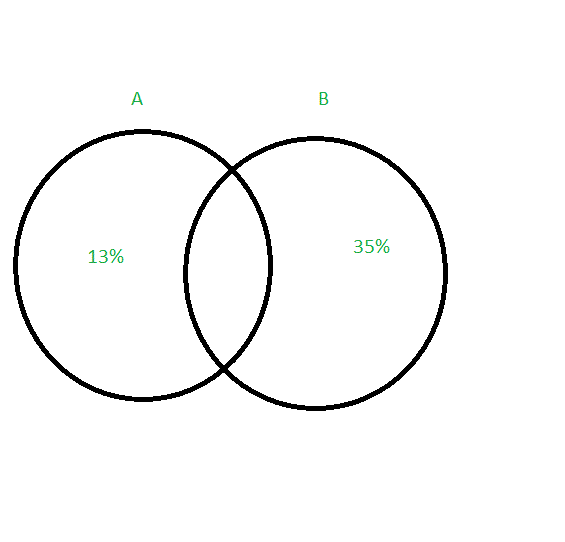The Venn diagram above represents the results from a recent survey given to middle school students. Category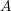represents the amount of students that only like pizza. Category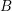represents the amount of students that only chicken nuggets.

What percentage of students only like pizza?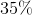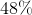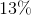Since Categoryrepresents the amount of students that only like pizza--and shows an amount of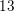percent in the Venn diagram, no calculations need to be performed.
Our answer is.